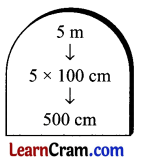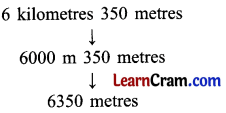# DAV Class 4 Maths Chapter 5 Worksheet 1 Solutions

The DAV Class 4 Maths Book Solutions and DAV Class 4 Maths Chapter 5 Worksheet 1 Solutions of Length offer comprehensive answers to textbook questions.

## DAV Class 4 Maths Ch 5 WS 1 Solutions

Question 1.
Fill in the blanks.
(a) 4m = _____ cm
(b) 8m = _____ cm
(c) 10km = _____ m
(d) 63km = _____ m
(a) 4m = 400 cm
(b) 8m = 800 cm
(c) 10km = 10,000 m
(d) 63km = 63,000 mQuestion 2.
Convert the following into centimetres
(a) 3m 40cm
3m 40cm
= 3 × 100cm + 40cm
= 300cm + 40cm
= 340 cm

(b) 19m 75cm
19 × 100cm + 75cm
= 1900cm + 75cm
= 1,975 cm

(c) 8m 3cm
8m 3 cm
= 8 × 100cm + 3cm
= 800cm + 3cm
= 803cm

(d) 34m 5m
34m 5m
= 34 × 100cm + 5cm
= 3400cm + 5cm
= 3,405cm

(e) 17m 30cm
17m 30cm
= 17 × 100cm + 30cm
= 1700cm + 30cm
= 1,730cm

(f) 50m 5cm
50m 5cm
= 50 × 100cm + 5cm
= 5000cm + 5 cm
= 5,005cm

Question 3.
Convert the following into metres.
(a) 4 km 315 m
(b) 7 km 125 m
(c) 25 km 500 m
(d) 19 km 5 m
(e) 152 km 35 m
(f) 4 km 8 m
(a) 4 km 315 m
= 4 × 1000 m + 315 m
= 4000 m + 315m
= 4,315m

(b) 7 km 125 m
= 7 × 1000 m + 125 m
= 7000 m + 125 m
= 7,125 m

(c) 25 km 500 m
= 25 × 1000 m + 500 m
= 25000 m + 500 m
= 25,500 m

(d) 19 km 5 m
= 19 × 1000 m + 5 m
= 19000 m + 5 m
= 19,005 m

(e) 152 km 35 m
= 152 × 1000 m + 35 m
= 15,200 m + 35 m
= 1,52,035 m

(f) 4 km 8 m
= 4 × 1000 m + 8 m
= 4000 m + 8 m
= 4,008 mQuestion 4.
State ‘True’ or ‘False’.
(a) 6 m 3 cm = 63 cm
(b) 14 m 20 cm = 1420 cm
(c) 9 km 52 m = = 952 m
(d) 2 km 2 m = 2002 m
(e) 26 km 516 m = 26516 m
(f) 10,000 m = 10 km
(a) False
(b) True
(c) False
(d) True
(e) True
(f) True

### DAV Class 4 Maths Chapter 5 Worksheet 1 Notes

• Length is measured in metre, centimetre and kilometre etc.
• The standard unit of length is metre. The smallest unit of length is millimetre (mm)
• 1 metre = 100 cm
• 1 kilometre = 1000 m
• To convert metre into centimetre and kilometre into metre, we multiply the number with 100 and 1,000 respectively.
• To convert centimetre into metre and metre into kilometre we divide the number with 100 and 1,000 respectively.
• For addition or subtraction of any given values, we first regroup them into their respective similar units.
For example, km is grouped with the value having km and metre is grouped with the value having metre. We never group m with km.

Review Exercise

Question 1.
Name any five things sold by a shop-keeper by measuring length.
Ribbon, water pipe, wire, cloth and wood ply etc.

Question 2.
Find the length of any three objects in your classroom.
1. Length of blackboard = 140 cm
2. Length of desk = 90 cm
3. Length of my pencil = 14.5 cm

Question 3.
Which unit will you choose to express the following:
(a) Height of a telephone pole.
(c) Distance between Delhi & Agra,
(d) Height of your study table.
(e) Distance between Earth & moon.
(a) m
(b) cm
(c) km
(d) m
(e) km
(f) kmConversions
Converting metre into centimetres
1 m = 100 cm
We multiply number of metres by 100 to convert ‘metres’ into centimetres.Converting kilometres into metres.
1 km = 1000 mConverting metres and centimetres into centimetres.
3 meters 70 centimetres into centimetres.We convert the number of ‘metres’ into ‘centimetres’ and then add it to the number of centimetres.

Converting kilometres and metres into metres.We convert the number of kilometres into metres and add it to the number of ‘metres’.